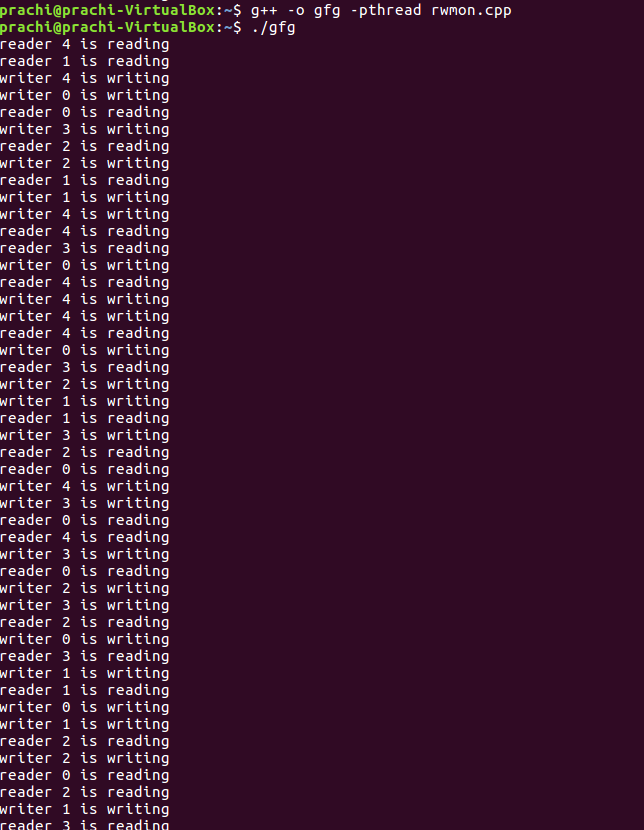• Difficulty Level : Hard
• Last Updated : 07 Feb, 2022

There is a shared resource that is accessed by multiple processes i.e. readers and writers. Any number of readers can read from the shared resource simultaneously, but only one writer can write to the shared resource at a time. When a writer is writing data to the resource, no other process can access the resource. A writer cannot write to the resource if there are any readers accessing the resource at that time. Similarly, a reader can not read if there is a writer accessing the resource or if there are any waiting writers.
The Reader-Writer problem using a monitor can be implemented using pthreads. The POSIX threads (or pthread) libraries are a standards-based thread API for C/C++. The library provides the following synchronization mechanisms:

• Mutexes (pthread_mutex_t) – Mutual exclusion lock:

The condition variable mechanism allows threads to suspend execution and relinquish the processor until some condition is true.

Execute the program using the following command in your Linux system

```\$g++ -pthread program_name.cpp
\$./a.out
or
\$./object_name```

Code:

## CPP

 `// Reader-Writer problem using monitors` `#include ``#include ``#include ``using` `namespace` `std;` `class` `monitor {``private``:``    ``// no. of readers``    ``int` `rcnt;` `    ``// no. of writers``    ``int` `wcnt;` `    ``// no. of readers waiting``    ``int` `waitr;` `    ``// no. of writers waiting``    ``int` `waitw;` `    ``// condition variable to check whether reader can read``    ``pthread_cond_t canread;` `    ``// condition variable to check whether writer can write``    ``pthread_cond_t canwrite;` `    ``// mutex for synchronization``    ``pthread_mutex_t condlock;` `public``:``    ``monitor()``    ``{``        ``rcnt = 0;``        ``wcnt = 0;``        ``waitr = 0;``        ``waitw = 0;` `        ``pthread_cond_init(&canread, NULL);``        ``pthread_cond_init(&canwrite, NULL);``        ``pthread_mutex_init(&condlock, NULL);``    ``}` `    ``// mutex provide synchronization so that no other thread``    ``// can change the value of data``    ``void` `beginread(``int` `i)``    ``{``        ``pthread_mutex_lock(&condlock);` `        ``// if there are active or waiting writers``        ``if` `(wcnt == 1 || waitw > 0) {``            ``// incrementing waiting readers``            ``waitr++;` `            ``// reader suspended``            ``pthread_cond_wait(&canread, &condlock);``            ``waitr--;``        ``}` `        ``// else reader reads the resource``        ``rcnt++;``        ``cout << "reader " << i << " is reading\n";``        ``pthread_mutex_unlock(&condlock);``        ``pthread_cond_broadcast(&canread);``    ``}` `    ``void` `endread(``int` `i)``    ``{` `        ``// if there are no readers left then writer enters monitor``        ``pthread_mutex_lock(&condlock);` `        ``if` `(--rcnt == 0)``            ``pthread_cond_signal(&canwrite);` `        ``pthread_mutex_unlock(&condlock);``    ``}` `    ``void` `beginwrite(``int` `i)``    ``{``        ``pthread_mutex_lock(&condlock);` `        ``// a writer can enter when there are no active``        ``// or waiting readers or other writer``        ``if` `(wcnt == 1 || rcnt > 0) {``            ``++waitw;``            ``pthread_cond_wait(&canwrite, &condlock);``            ``--waitw;``        ``}``        ``wcnt = 1;``        ``cout << "writer " << i << " is writing\n";``        ``pthread_mutex_unlock(&condlock);``    ``}` `    ``void` `endwrite(``int` `i)``    ``{``        ``pthread_mutex_lock(&condlock);``        ``wcnt = 0;` `        ``// if any readers are waiting, threads are unblocked``        ``if` `(waitr > 0)``            ``pthread_cond_signal(&canread);``        ``else``            ``pthread_cond_signal(&canwrite);``        ``pthread_mutex_unlock(&condlock);``    ``}` `}` `// global object of monitor class``M;` `void``* reader(``void``* id)``{``    ``int` `c = 0;``    ``int` `i = *(``int``*)id;` `    ``// each reader attempts to read 5 times``    ``while` `(c < 5) {``        ``usleep(1);``        ``M.beginread(i);``        ``M.endread(i);``        ``c++;``    ``}``}` `void``* writer(``void``* id)``{``    ``int` `c = 0;``    ``int` `i = *(``int``*)id;` `    ``// each writer attempts to write 5 times``    ``while` `(c < 5) {``        ``usleep(1);``        ``M.beginwrite(i);``        ``M.endwrite(i);``        ``c++;``    ``}``}` `int` `main()``{``    ``pthread_t r, w;``    ``int` `id;``    ``for` `(``int` `i = 0; i < 5; i++) {``        ``id[i] = i;` `        ``// creating threads which execute reader function``        ``pthread_create(&r[i], NULL, &reader, &id[i]);` `        ``// creating threads which execute writer function``        ``pthread_create(&w[i], NULL, &writer, &id[i]);``    ``}` `    ``for` `(``int` `i = 0; i < 5; i++) {``        ``pthread_join(r[i], NULL);``    ``}``    ``for` `(``int` `i = 0; i < 5; i++) {``        ``pthread_join(w[i], NULL);``    ``}``}`

Output:My Personal Notes arrow_drop_up